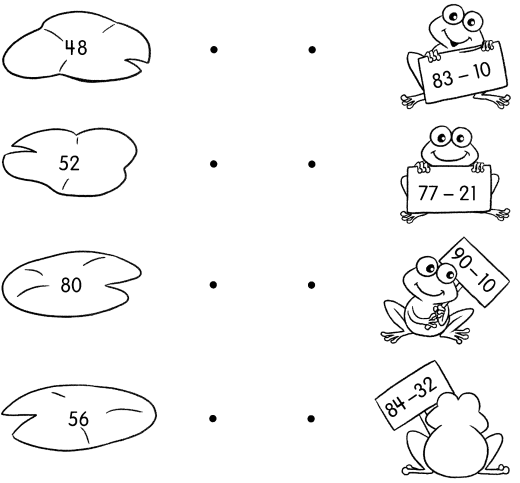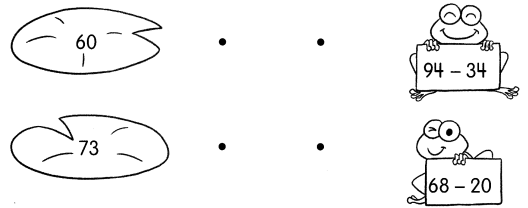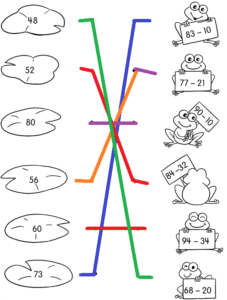# Math in Focus Grade 1 Chapter 17 Practice 3 Answer Key Subtraction Without Regrouping

Go through the Math in Focus Grade 1 Workbook Answer Key Chapter 17 Practice 3 Subtraction Without Regrouping to finish your assignments.

## Math in Focus Grade 1 Chapter 17 Practice 3 Answer Key Subtraction Without Regrouping

Count back to subtract.

Example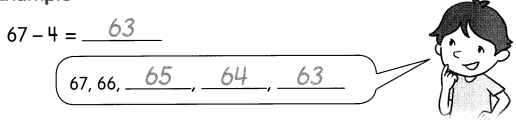Question 1.
95 – 3 = ____________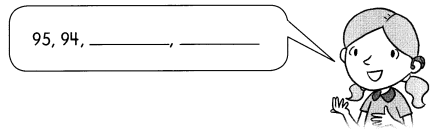95 – 3 = 92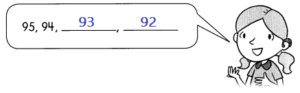Explanation:
By subtracting smaller number from bigger number, we get the difference.
95 – 3 = 92
Count back to subtract or 3 numbers.
92 , 93 , 94 , 95 are the numbers

Question 2.
88 – 5 = _____________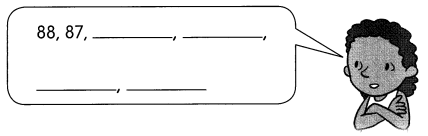88 – 5 = 83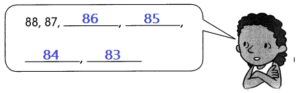Explanation:
By subtracting smaller number from bigger number, we get the difference.
88 – 5 = 83
Count back to subtract or 5 numbers.
83, 84, 85, 86, 87, 88 are the numbers.

Question 3.
79 – 6 = _____________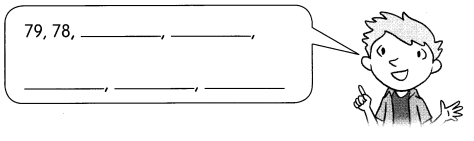79 – 6 = 73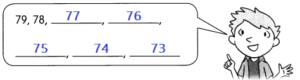Explanation:
By subtracting smaller number from bigger number, we get the difference.
79 – 6 = 73
Count back to subtract or 6 numbers.
73, 74, 75, 76, 77, 78, 79 are the numbers.

Subtract.

Question 4.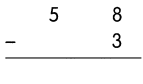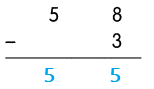Explanation:
because the numbers can be easily deducted since the top number is always larger than the bottom number.
So, 58 – 3 = 55

Question 5.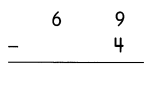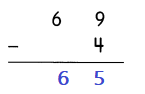Explanation:
because the numbers can be easily deducted since the top number is always larger than the bottom number.
So, 69 – 4 = 65

Question 6.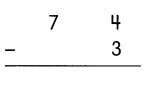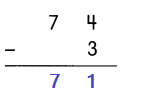Explanation:
because the numbers can be easily deducted since the top number is always larger than the bottom number.
So, 74 – 3 = 71

Question 7.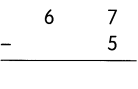Explanation:
because the numbers can be easily deducted since the top number is always larger than the bottom number.
So, 67 – 5 = 62

Question 8.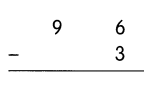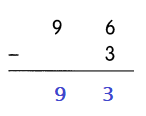Explanation:
because the numbers can be easily deducted since the top number is always larger than the bottom number.
So, 96 – 3 = 93

Question 9.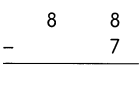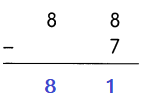Explanation:
because the numbers can be easily deducted since the top number is always larger than the bottom number.
So, 88 – 7 = 81

Fill in the missing numbers.

Question 10.
79 – 6 = ___________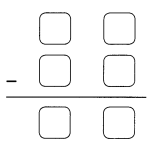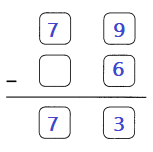Explanation:
You need to place the subtrahend below the minuend so that the ones-place numbers fall in the same column.
Now you have to subtract each column separately and in order, starting with the ones-place column.
Then place the answer of the subtractions below each one of the columns.
So, 79 – 6 = 73

Question 11.
99 – 5 = _____________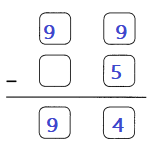Explanation:
You need to place the subtrahend below the minuend so that the ones-place numbers fall in the same column.
Now you have to subtract each column separately and in order, starting with the ones-place column.
Then place the answer of the subtractions below each one of the columns.
So, 99 – 5 = 94

Subtract.

Question 12.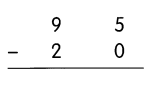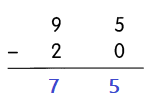Explanation:
You need to place the subtrahend below the minuend so that the ones-place numbers fall in the same column.
Now you have to subtract each column separately and in order, starting with the ones-place column, tens and so on…
The last step is to place the answer of the subtractions below each one of the columns, always put it in order.
So, 95 – 20 = 75

Question 13.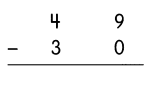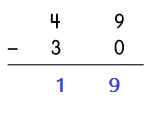Explanation:
You need to place the subtrahend below the minuend so that the ones-place numbers fall in the same column.
Now you have to subtract each column separately and in order, starting with the ones-place column, tens and so on…
The last step is to place the answer of the subtractions below each one of the columns, always put it in order.
So, 49 – 30 = 19

Question 14.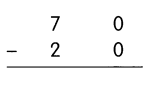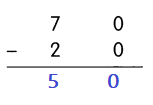Explanation:
You need to place the subtrahend below the minuend so that the ones-place numbers fall in the same column.
Now you have to subtract each column separately and in order, starting with the ones-place column, tens and so on…
The last step is to place the answer of the subtractions below each one of the columns, always put it in order.
So, 70 – 20 = 50

Question 15.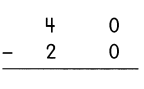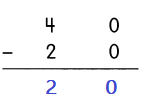Explanation:
You need to place the subtrahend below the minuend so that the ones-place numbers fall in the same column.
Now you have to subtract each column separately and in order, starting with the ones-place column, tens and so on…
The last step is to place the answer of the subtractions below each one of the columns, always put it in order.
So, 40 – 20 = 20

Question 16.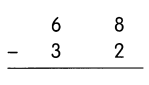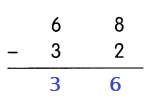Explanation:
You need to place the subtrahend below the minuend so that the ones-place numbers fall in the same column.
Now you have to subtract each column separately and in order, starting with the ones-place column, tens and so on…
The last step is to place the answer of the subtractions below each one of the columns, always put it in order.
So, 68 – 32 = 36

Question 17.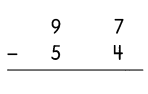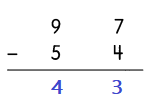Explanation:
You need to place the subtrahend below the minuend so that the ones-place numbers fall in the same column.
Now you have to subtract each column separately and in order, starting with the ones-place column, tens and so on…
The last step is to place the answer of the subtractions below each one of the columns, always put it in order.
So, 97 – 54 = 43

Fill in the missing numbers.

Question 18.
56 – 23 = _________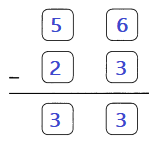Explanation:
Place the subtrahend below the minuend so that the ones-place numbers fall in the same column.
Now subtract each column separately and in order, starting with the ones-place column, tens and so on…
Then place the answer of the subtractions below each one of the columns, always put it in order.
So, 56 – 23 = 33

Question 19.
86 – 42 = ___________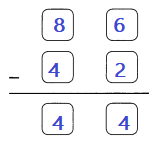Explanation:
Place the subtrahend below the minuend so that the ones-place numbers fall in the same column.
Now subtract each column separately and in order, starting with the ones-place column, tens and so on…
Then place the answer of the subtractions below each one of the columns, always put it in order.
So, 86 – 42 = 44

Match

Question 20.